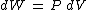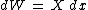# Thermodynamic processes

## Thermodynamic processes

Changes of any property of an aggregation of matter and energy, accompanied by thermal effects.

#### Systems and processes

To evaluate the results of a process, it is necessary to know the participants that undergo the process, and their mass and energy. A region, or a system, is selected for study, and its contents determined. This region may have both mass and energy entering or leaving during a particular change of conditions, and these mass and energy transfers may result in changes both within the system and within the surroundings which envelop the system.

To establish the exact path of a process, the initial state of the system must be determined, specifying the values of variables such as temperature, pressure, volume, and quantity of material. The number of properties required to specify the state of a system depends upon the complexity of the system. Whenever a system changes from one state to another, a process occurs.

The path of a change of state is the locus of the whole series of states through which the system passes when going from an initial to a final state. For example, suppose a gas expands to twice its volume and that its initial and final temperatures are the same. An extremely large number of paths connect these initial and final states. The detailed path must be specified if the heat or work is to be a known quantity; however, changes in the thermodynamic properties depend only on the initial and final states and not upon the path. A quantity whose change is fixed by the end states and is independent of the path is a point function or a property.

#### Pressure-volume-temperature diagram

Whereas the state of a system is a point function, the change of state of a system, or a process, is a path function. Various processes or methods of change of a system from one state to another may be depicted graphically as a path on a plot using thermodynamic properties as coordinates.

The variable properties most frequently and conveniently measured are pressure, volume, and temperature. If any two of these are held fixed (independent variables), the third is determined (dependent variable). To depict the relationship among these physical properties of the particular working substance, these three variables may be used as the coordinates of a three-dimensional space. The resulting surface is a graphic presentation of the equation of state for this working substance, and all possible equilibrium states of the substance lie on this P-V-T surface.

Because a P-V-T surface represents all equilibrium conditions of the working substance, any line on the surface represents a possible reversible process, or a succession of equilibrium states.

The portion of the P-V-T surface shown in Fig. 1 typifies most real substances; it is characterized by contraction of the substance on freezing. Going from the liquid surface to the liquid-solid surface onto the solid surface involves a decrease in both temperature and volume. Water is one of the few exceptions to this condition; it expands upon freezing, and its resultant P-V-T surface is somewhat modified where the solid and liquid phases abut.

One can project the three-dimensional surface onto the P-T plane as in Fig. 2. The triple point is the point where the three phases are in equilibrium. When the temperature exceeds the critical temperature (at the critical point), only the gaseous phase is possible.

#### Temperature-entropy diagram

Energy quantities may be depicted as the product of two factors: an intensive property and an extensive one. Examples of intensive properties are pressure, temperature, and magnetic field; extensive ones are volume, magnetization, and mass. Thus, in differential form, work is the product of a pressure exerted against an area which sweeps through an infinitesimal volume, as in Eq. (1).

(1)As a gas expands, it is doing work on its environment. However, a number of different kinds of work are known. For example, one could have work of polarization of a dielectric, of magnetization, of stretching a wire, or of making new surface area. In all cases, the infinitesimal work is given by Eq. (2),
(2)where X is a generalized applied force which is an intensive quantity, and dx is a generalized displacement of the system and is thus extensive.

By extending this approach, one can depict transferred heat as the product of an intensive property, temperature, and a distributed or extensive property defined as entropy, for which the symbol is S. See Entropy

#### Reversible and irreversible processes

Not all energy contained in or associated with a mass can be converted into useful work. Under ideal conditions only a fraction of the total energy present can be converted into work. The ideal conversions which retain the maximum available useful energy are reversible processes.

Characteristics of a reversible process are that the working substance is always in thermodynamic equilibrium and the process involves no dissipative effects such as viscosity, friction, inelasticity, electrical resistance, or magnetic hysteresis. Thus, reversible processes proceed quasistatically so that the system passes through a series of states of thermodynamic equilibrium, both internally and with its surroundings. This series of states may be traversed just as well in one direction as in the other.

Actual changes of a system deviate from the idealized situation of a quasistatic process devoid of dissipative effects. The extent of the deviation from ideality is correspondingly the extent of the irreversibility of the process. See Thermodynamic principles

References in periodicals archive ?
This book outlines recent advances in the theoretical study of the collection of basic physical and thermodynamic processes in the troposphere, stratosphere, and mesosphere.
These thermodynamic processes are typical of renewable energy transformers, cogeneration units, heat pumps, heat exchangers, energy accumulators, working fluid circulation pumps as well as control and adjustment equipment and others.
In order to properly reflect the mechanical response of the system, it is analysed in terms of the mechanical and thermodynamic processes.
The engine uses mechanical and thermodynamic processes to reject this heat, and the core components have to be produced to a high degree of precision and smoothness.
Contract notice: Research basal phenomena light-emitting semiconductor, optical, electrical and thermodynamic processes and their introduction into practical application through adaptive technology.
Paolo Franceschetti underlines our technologies which are based on the optimization of thermodynamic processes needed a Company able to guarantee their industrialization and sales exporting this green technology all over the World.
Professor Ding said: "My research will cover materials, thermodynamic processes and cycles, storage components and devices, system integration and optimisation, and applications of cryogenic energy storage.
They hypothesize that the degradation of machinery components is a consequence of irreversible thermodynamic processes, that is, a time-dependent phenomenon with increasing disorder.
These bin zones are bound by the capability of each technology's thermodynamic processes to achieve supply air in the ASHRAE recommended or allowable environmental envelopes for IT equipment.
of Texas) discusses the foundations of equilibrium and nonequilibrium statistical physics and concentrates on the universal nature of thermodynamic processes.
Investigation of the Thermodynamic Processes of Expander by Means of a P-[theta] Diagram
Coil geometry was found by simulating transient of electromagnetic, magneto mechanical ant thermodynamic processes using Matlab[R] Simulink[R] and Matlab[R] software.

Site: Follow: Share:
Open / Close